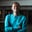Related Tags

php
communitycreator

# What are PHP arrow functions?Zubair Idris Aweda

Arrow functions are introduced as an update in the PHP version 7.4. Arrow functions are supposed to be a more concise version of anonymous functions. Arrow functions can be seen as shorthand functions that automatically inherit the parent scope’s variables. This is an advantage arrow functions have over regular functions and even anonymous functions.

### Syntax

Arrow functions generally have the following syntax:

fn (arguments) => expression


Arrow functions always have to start with the fn keyword and can only have one expression. The expression’s output is returned. Arrow functions are only to be used for simple operations.

### Code

#### Example 1

For example, you can use arrow function syntax to define and use a function to sum up numbers as follows:

<?php

$add = fn ($a, $b) =>$a + $b; echo$add(4, 5);

Arrow function

You can use anonymous functions to create the same function normally as follows:

<?php

function add($a,$b)
{
return $a +$b;
}

Regular function

#### Example 2

In the example below, the arrow function uses a variable outside its scope and a regular function throws an error.

<?php

$greeting = 'Welcome to Educative, master '; // variable in parent scope$greet = fn ($username) =>$greeting . $username; // arrow function echo "arrow function: ",$greet('John')  . "\n"; // print result of  arrow function

function longGreet($username) // regular function { return$greeting . $username; } echo "regular function: ", longGreet('James') . "\n"; // print result of regualr function  #### Example 3 You can also pass arrow functions as closure to other functions. For example, to get the square of every item in an array, use the code below: <?php$nums = [2, 3, 4, 9, 12];

$squareNums = array_map(fn ($num) => $num *$num, $nums); print_r($squareNums);


RELATED TAGS

php
communitycreator

CONTRIBUTORZubair Idris Aweda
RELATED COURSES

View all Courses

Keep Exploring

Learn in-demand tech skills in half the time